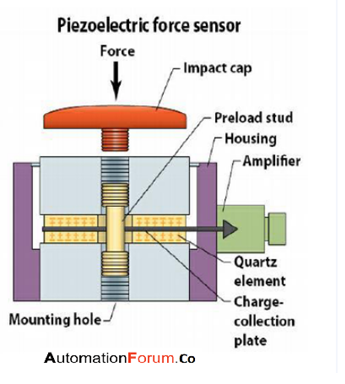# What is a piezoelectric transducer and how does it workWhat is a transducer

A transducer is a device that can convert one form of energy to other, transducers are classified into many types some of them are electrical, magnetic, and piezoelectric.

What is a piezoelectric effect

In a piezoelectric material, if we apply a mechanical force it could change the dimension of the material. Because of this, an electric potential will appear across certain surfaces of a crystal. This potential is produced by displacement of charges, this effect is known as the piezoelectric effect.

What is a piezoelectric transducer and how does it work

A piezoelectric transducer is made up of special kind of material, it is made up of piezoelectric. This material has a specialty that if the material is subjected to pressure it produces an EMF this EMF can be measured to find out the applied pressure. Elements that exhibit piezoelectric properties are called electro-resistive elements. Piezoelectric materials are divided into two categories they are the natural and synthetic groups. The natural group consists of quartz and Rochelle salt, while the synthetic group consists of lithium sulphate, and ethylene diamine tartarate.  The working principle of this transducer can be explained as when a force is applied on the quartz crystal an electric charge will be produced on the crystal surface and the charge thus produced can be called piezoelectricity. The charge produced will be proportional to the applied pressure.

What is the principle of piezoelectric transducer

Depending on how a piezoelectric material is cut, three main modes of operation can be distinguished they are transverse, longitudinal, and shear.

Transverse effect

When a force is applied along a neutral axis ‘y’ then it generates charges along the ‘x’ direction, perpendicular to the line of force. The charge is depended upon the geometrical dimensions of the piezoelectric element.

Longitudinal effect

The amount of charge produced is proportional to the applied force and it is independent of the size and shape of the piezoelectric element.

Shear effect

The charge produced is proportional to the applied forces and they are independent of the size and shape of the element.What is a piezoelectric sensor and where is it used

The piezoelectric sensor works on the principle of piezoelectric effect, thus it can measure the changes in acceleration, pressure, temperature, etc. The measurement is done by converting it into an electric charge. Piezoelectric pressure sensor measures dynamic pressure, they are not suited for static pressure measurements. A piezoelectric sensor is an active sensor and it does not need the help of external power as it is self-generating.

What are the materials used for piezoelectric transducer

The materials which are used for the measurement process must have certain properties like stability, high output, insensitive to extreme temperature and humidity and it must have the ability to formed or machined into any shape. Quartz is mostly used as the piezoelectric material because of its stability. This material is mostly shaped into a rectangular or square plate and it would be held between two electrodes. Crystal is connected to the appropriate electronic circuit to obtain the proper output.

How can we categorize the piezoelectric transducer

A piezoelectric transducer can be categorized into sonic, ultrasonic and mega-sonic transducers based on the operating frequency. In all of this category, the design and function of transducer vary significantly. The sonic transducer works at an audible frequency range which would be less than 20 Khz. Examples of sonic transducers are buzzer and unimorph transducer. The ultrasonic transducers usually work at a frequency range from 20-200 kHz. The mega sonic transducers work at the frequency range of Mhz.

What are the advantages of piezoelectric transducer

• These transducers have a high-frequency response so that it can measure parameters which change at high speed
• It has a high transient response, which means that it can detect the events at microseconds and can also give the linear output
• High output can be measured by the help of electronic circuit
• They are small in size and have rugged construction.

What are the disadvantages of piezoelectric transducer

• The output of the piezoelectric transducer is very low, so an external electronic circuit has to be connected
• The piezoelectric transducer has high impedance so they have to be connected to the amplifier and the auxiliary circuit.
• It is very difficult to shape the crystals with sufficient strength

What are the applications of piezoelectric transducer

• It is used as an accelerometer, because of its excellent frequency response
• It can be used to measure force, pressure, and displacement in terms of voltage
• It is designed to use as a pre-tensional bolt so that both tensional and compression force measurements can be made
• It is useful in military field for depth sounders, sonar, and hydrophones
• It is useful in the medical field for ultrasonic imaging
• It is very useful for dynamic measurements
• It is used in the automotive industry, in airbag sensor, airflow sensor, and knock sensor
Scroll to Top